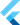# Iterators: understanding and creating your own

on Sunday, 26th of July, 2020

An `iterator` is an interface for getting items, one at a time, from an object. All `Iterable` types have iterators. Iterators, in reality, are just objects that tell Dart in what order to access objects one at a time. This is what allows us to use language features like `for in` loops. This all feels very hand-wavy to type, so let's look at some examples to show you what I mean.

The code example will probably make the most sense if you first understand what the goal is.

Suppose you want to make a `Grid` class. The grid class will represent data that looks like this:

``````[
[ 0, 1, 2],
[ 3, 4, 5],
[ 6, 7, 8],
]``````

So, it's just a list of lists. Nothing special, until you want to be able to do this on it:

``````for (var point in grid) {
print(point);
}

// and you expect that to print this:
// => 0
// => 1
// => 2
// => 3
// => 4
// => 5
// => 6
// => 7
// => 8``````

In otherwords, you want it to be smart enough to wrap to the next line when you hit the end of any given horizontal like. That'd be pretty neat. This (large) code example shows how you could achieve that:

I explain the code below, if you'd rather see the explanation first.

```    ```

class Point <T> {
final int x;
final int y;
final T value;

Point(this.x, this.y, this.value);

String toString() => "(\$x, \$y), value: \$value";
}

class Grid<T> with IterableMixin<Point<T>> {
List<List<Point>> _grid;

Grid() : _grid = <List<Point>>[];

factory Grid.fromNestedList(List<List<T>> grid) {
final _new = <List<Point<T>>>[];
for (var i = 0; i < grid.length; i++) {
_new.add(<Point<T>>[]);
for (var j = 0; j < grid.length; j++) {
_new[i].add(Point<T>(i, j, grid[i][j]));
}
}

return Grid().._grid = _new;
}

@override
Iterator<Point<T>> get iterator => GridIterator(_grid);
}

class GridIterator<T> extends Iterator<Point<T>> {
GridIterator(this._grid) {
_crossAxisCount = _grid.length - 1;
_verticalAxisCount = _grid.length - 1;
}

int _iIndex = -1;
int _jIndex = -1;

int _crossAxisCount;
int _verticalAxisCount;
final List<List<Point>> _grid;

@override
Point<T> get current {
if (_iIndex < 0) return null;
if (_jIndex < 0) return null;
if (_iIndex > _crossAxisCount) return null;
if (_jIndex > _verticalAxisCount) return null;
return _grid[_jIndex][_iIndex];
}

@override
bool moveNext() {
if (_iIndex < _crossAxisCount) {
_iIndex++;
} else {
if (_jIndex <= _verticalAxisCount) {
_iIndex = 0;
_jIndex++;
}
}

if (_jIndex > _verticalAxisCount) {
return false;
}

return true;
}
}

void main() {
Grid grid = Grid.fromNestedList([
[1, 2, 3],
[4, 5, 6],
[7, 8, 9]
]);

for (var point in grid) {
print(point);
}
}
``````

Important pieces of the code:

• Make a class that `extends IterableMixin`. This forces the class to implement a getter called `iterator`. That iterator must return an... `Iterator`.
• Next, create a class that implements that extends that `Iterator`. This class must contain two methods:
• `T current()`
• this should return the current element in your class in any given iteration of a loop.
• for example, when you write `for (var point in grid)`, `point` is what is returned by `Iterator.current`.
• `bool moveNext`
• this is called internally at the end of every iteration of a loop. It determines what the next `current` is.

This code may seem complex (because it is), but boy howdy do I encourage you to really get it. If you understand this, it really makes you start realizing that you're fully capable of understand how many features of Dart are implemented under the hood.

Its important to note that you don't have to use iterables under the hood in the class that you're making iterable. You could do this if you really wanted:

``````class User extends IterableMixin<dynamic> {
final String name;
final int age;
final String address;
final String username;
// etc
}

/// and then write the iterator class, which I'm skipping
/// and eventually have functionality like this:

for (var prop in user) {
print(prop)
}

// prints
// 'Eric'
// 29
// 'Portland OR'
// 'ericwindmill'``````

To be sure, I don't know why you would want to do that, but you certainly could write an iterator that did this. Because, to recap, all an iterator really does is give us a way to visit elements of an object one at a time, in a specific order.